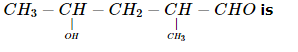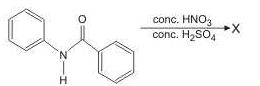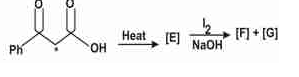# WBJEE Chemistry Test - 14

## 40 Questions MCQ Test WBJEE Sample Papers, Section Wise & Full Mock Tests | WBJEE Chemistry Test - 14

Description
Attempt WBJEE Chemistry Test - 14 | 40 questions in 60 minutes | Mock test for JEE preparation | Free important questions MCQ to study WBJEE Sample Papers, Section Wise & Full Mock Tests for JEE Exam | Download free PDF with solutions
QUESTION: 1

Solution:
QUESTION: 2

Solution:
QUESTION: 3

### When HNO₃ is dropped into the palm and washed with water, it turns yellow. It shows the presence of

Solution:
QUESTION: 4
Schiff's reagent gives pink colour with
Solution:
QUESTION: 5
The energy librated per mole of H⁺ ion by neutralisation of strong acid and strong base :
Solution:
QUESTION: 6

The maximum number of 90angles between bond pair-bond pair of electrons is observed in

Solution:
QUESTION: 7
Potassium chromate solution is added to an aqueous solution of a metal chloride. The precipitate thus obtained are insoluble in acetic acid. These are subjected to flame test, the colour of the flame is
Solution:
QUESTION: 8
An example of water soluble vitamin is
Solution:
QUESTION: 9
Which of the following pair of ions have same paramagnetic moment?
Solution:
QUESTION: 10
The alkyl halide is converted into ethyl alcohol by
Solution:
QUESTION: 11
A chemical reaction is in equilibrium when
Solution:
QUESTION: 12
Which of the following pair is wrong?
Solution:
QUESTION: 13
The reaction is spontaneous if the cell potential is
Solution:
QUESTION: 14
The IUPAC name of (CH₃)₃ C - CH = CH₂ is
Solution:
QUESTION: 15
Which of the following is the function of aspirin?
Solution:
QUESTION: 16
When the product of pressure and volume is plotted against pressure for a given amount of the gas, the line obtained is
Solution:
QUESTION: 17
When AgNO₃ is added to a solution of Co(NH₃)₅ Cl₃, the precipitate of AgCl shows two ionizable chloride ions. This means:
Solution:
QUESTION: 18
The nitride salt of Ca when treated with H₂O gives
Solution:
QUESTION: 19
Aluminium (III) chloride forms a dimer because
Solution:
QUESTION: 20
Phosphorus is manufactured by heating in furnace
Solution:
QUESTION: 21
The principal constitutent of pyrex glass is
Solution:
QUESTION: 22
A metal which does not liberate H₂(g) from acids is;
Solution:
QUESTION: 23
How many structural isomers could be obtained from alkalne C₆H₁₄?
Solution:
QUESTION: 24
Isotopes of elements were discovered by
Solution:
QUESTION: 25
Which of the following will exhibit tautomerism?
Solution:
QUESTION: 26
WHich of the following polymer is an example of fibre?
Solution:
QUESTION: 27
Which of the following is a bio-degradable polymer?
Solution:
QUESTION: 28
In which of the following reaction there is no change in oxidation number
Solution:
QUESTION: 29

AB is an ionic solid. If the ratio of ionic radii of A+ and B is 0 .52. What is the coordination number of B

Solution:

Ratio r +/r-  = 0.414 - 0.732
Coordination no. is 6.

QUESTION: 30
A pair of diastereoisomers will be formed when :
Solution:
QUESTION: 31

The IUPAC name ofSolution:
QUESTION: 32

Which one of the following compounds will be most easily attacked by an electrophile ?

Solution:
QUESTION: 33

The number of stereoisomers possible for a compound of the molecular formula
CH3― CH ═ CH ― CH (OH)   ― Me is

Solution:
QUESTION: 34

In the following reaction,the structure of the major product 'X' is

Solution:
QUESTION: 35

In the following reaction sequence, the correct structures of E, F and G are(* implies 13C labelled carbon)

Solution:
QUESTION: 36

Which statement(s) about conductivity of water is/are correct?

Solution:
QUESTION: 37

Nitrogen (I) oxide is produced by :

Solution:
QUESTION: 38

Sodium sulphate is highly soluble in water, but calcium sulphate is only sparingly soluble because

Solution:
QUESTION: 39

Which of the following statements are not correct ?

Solution:
QUESTION: 40

Statements that are the true among the following are:

Solution:Use Code STAYHOME200 and get INR 200 additional OFF Use Coupon Code